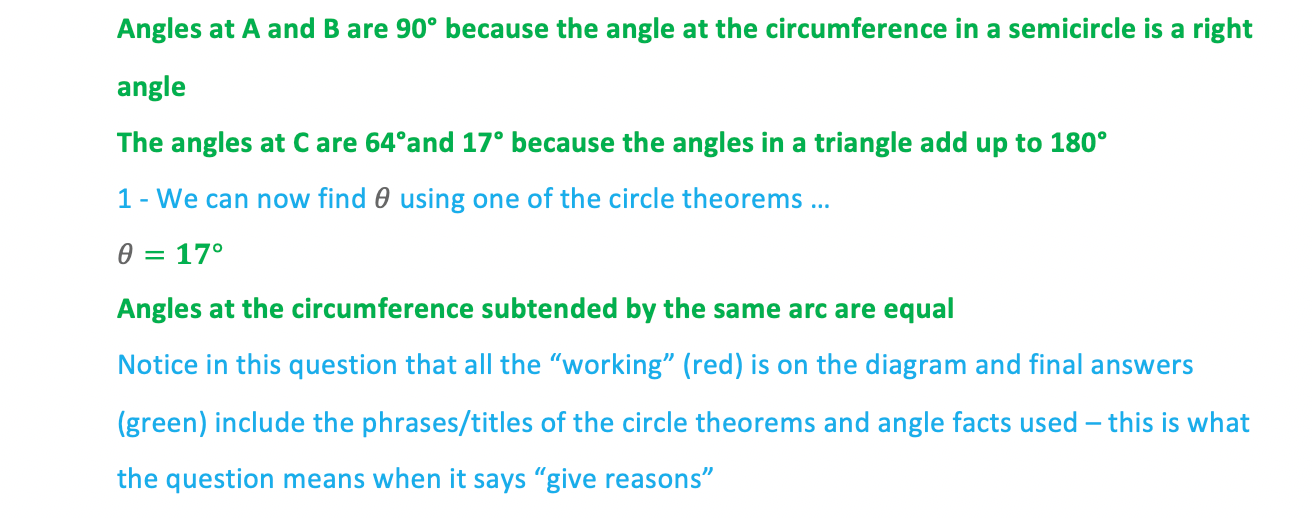# Edexcel IGCSE Maths 复习笔记 4.6.1 Circle Theorems - Angles at Centre & Circumference

Edexcel IGCSE Maths 复习笔记 4.6.1 Circle Theorems - Angles at Centre & Circumference

#### What are circle theorems?

• You will have learned a lot of angle facts for your GCSE – angles in polygons, angles with parallel lines
• Circle Theorems deal with angle facts that occur with shapes and lines drawn within and connected to a circle

#### What do I need to know?

• Be familiar with the names of parts of a circle and lines within and outside of a circle: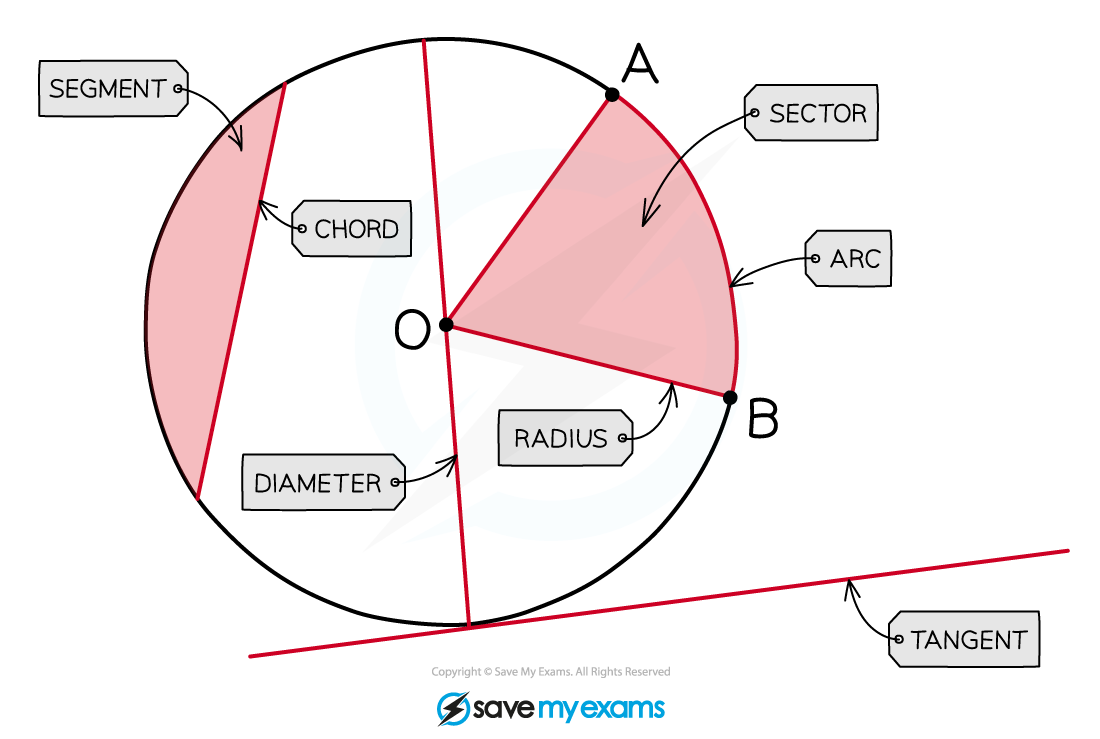• To solve hard problems you may need to use the angle facts you are already familiar with from triangles, polygons, and parallel lines
• You may also have to use circumference and area formulas, so ensure you’re familiar with:

C = πdand

A = πr2

#### The first 3 circle theorems

1. The angle at the circumference in a semicircle is a right angle• This theorem should be self-explanatory form its name/title
• The semicircle arises if you ignore the right-hand side of the diameter in the diagram above
• Look out for triangles hidden among other lines/shapes within the circle

2. Angles at the circumference subtended by the same arc are equal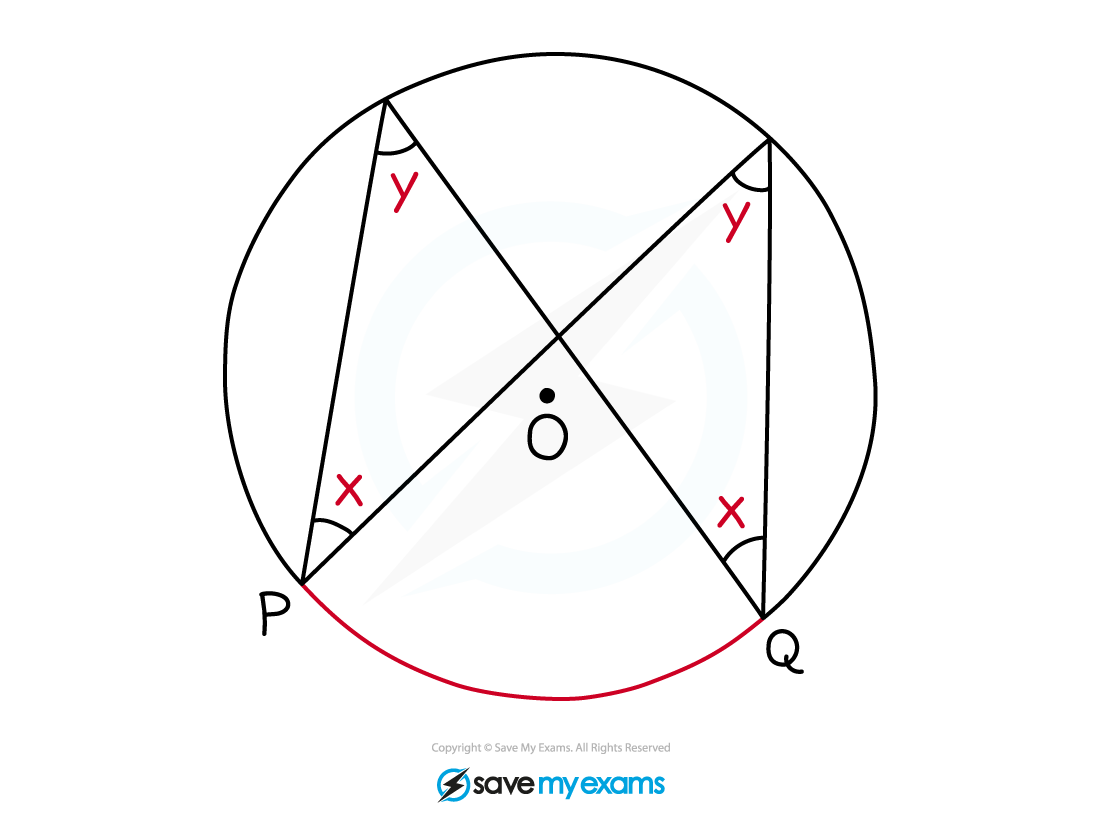• Subtended means the equal angles are created by drawing chords from the ends of the arc PQ
• Theses chords may or may not pass through the centre
• Both pairs of angles are equal

3. Angle subtended by an arc at the centre is twice the angle at the circumference• Similar to above, the chords (radii) to the centre and the chords to the circumference are both drawn from (subtended by) the ends of the arc PQ
• This theorem can also happen when the ‘triangle parts’ overlap: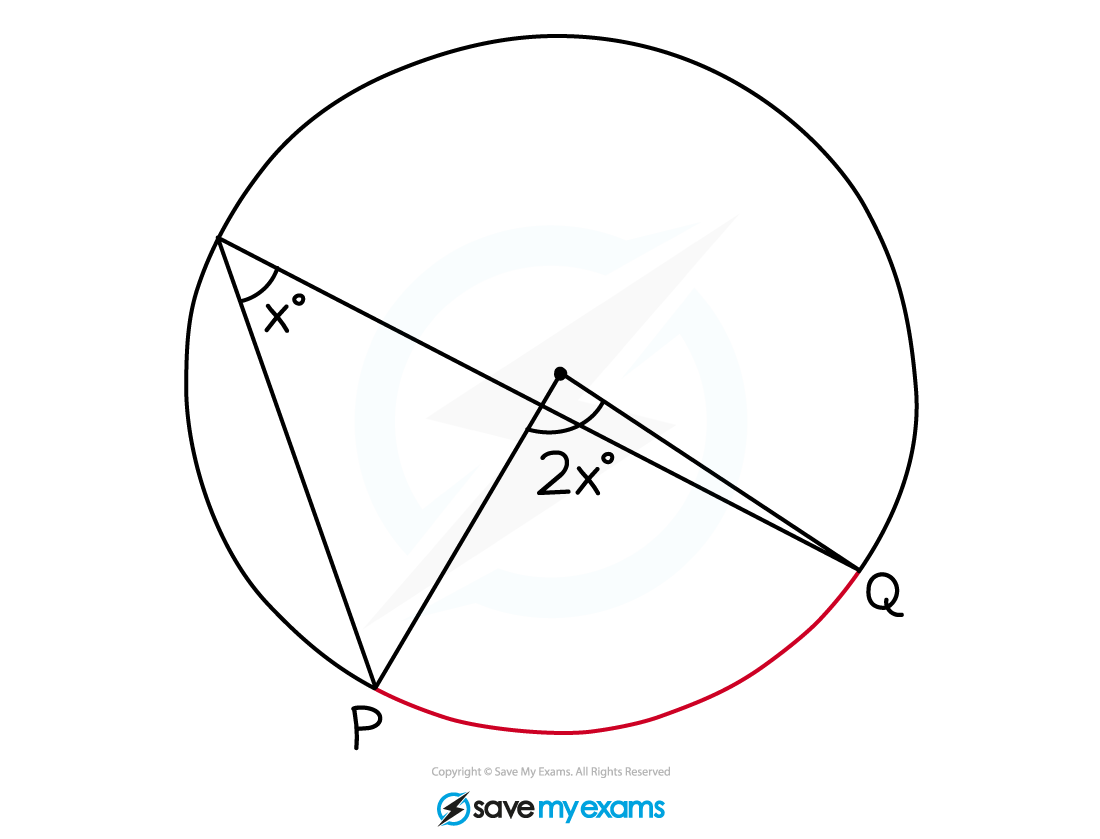#### Exam Tip

Add anything you can to a diagram you have been given – write in any angles and lengths you can work out, even if they don’t seem relevant to the actual question.For each angle you work out, try to assign an angle fact or circle theorem to it – questions often ask for “reasons” and the names/titles/phrases for each of these is exactly what they are after.When asked to “give reasons” aim to quote an angle fact or circle theorem for every angle you find, not just one for the final answer.

#### Worked Example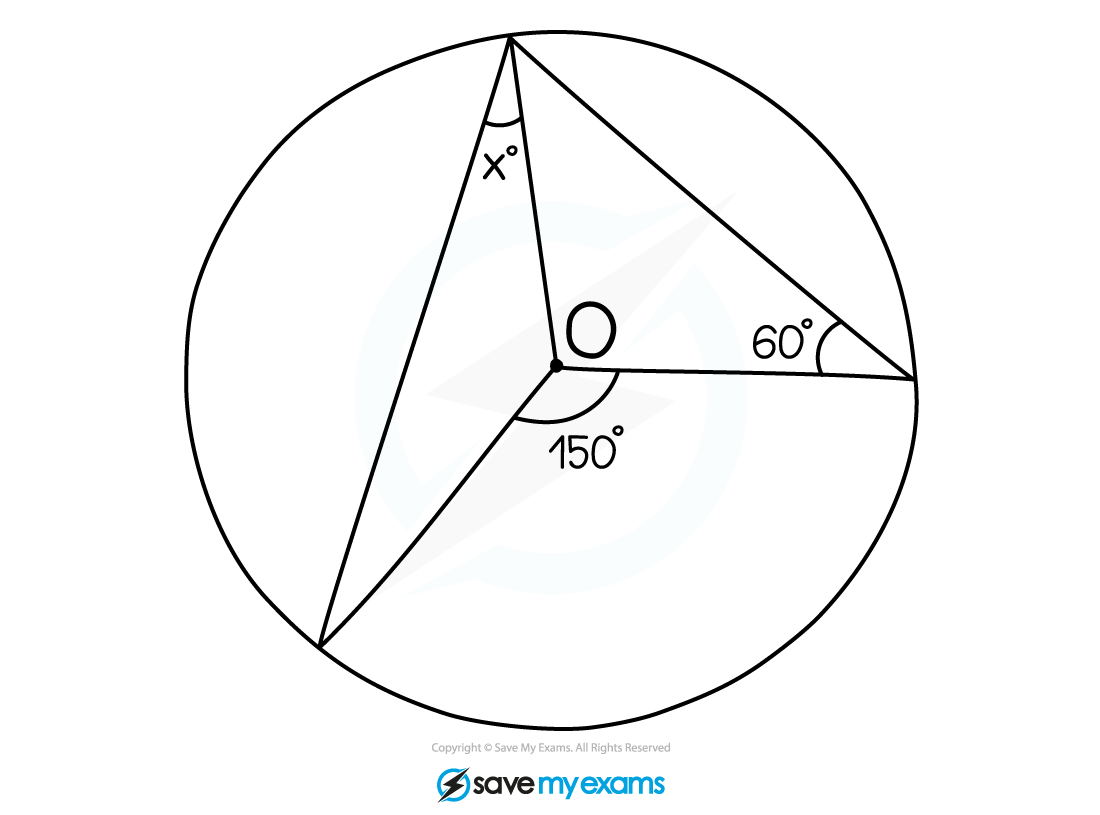#### Worked Example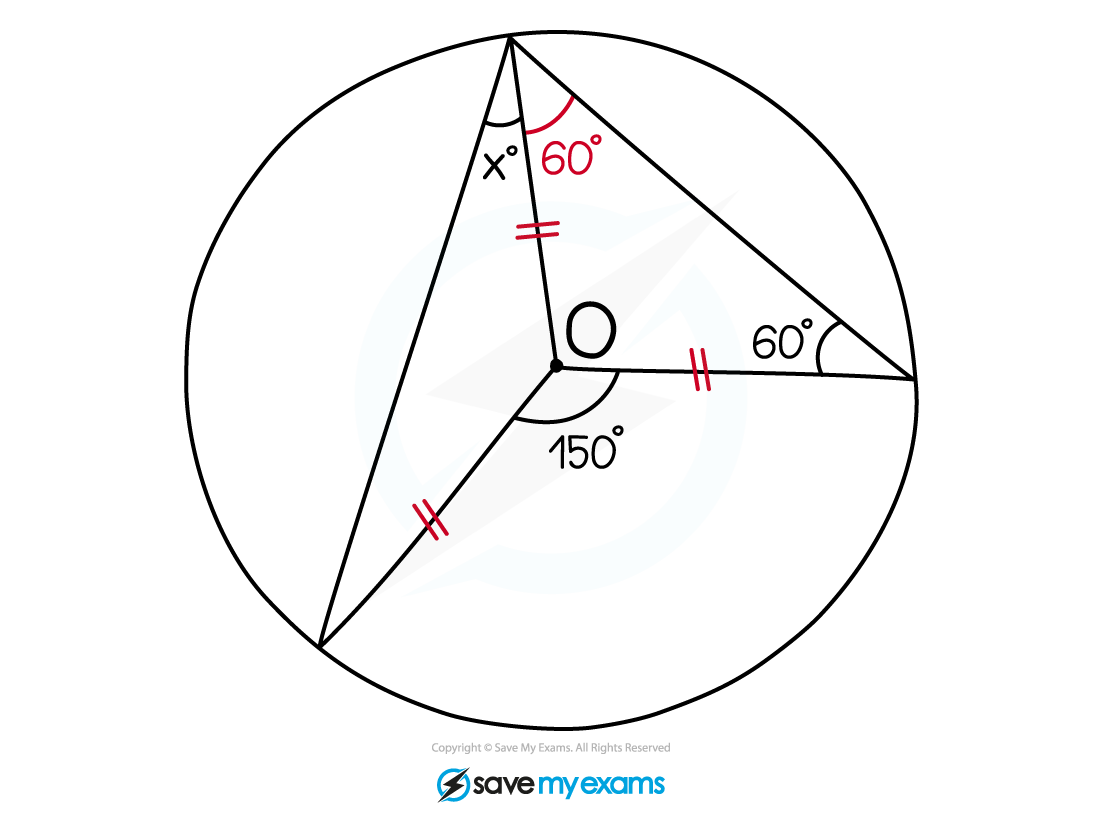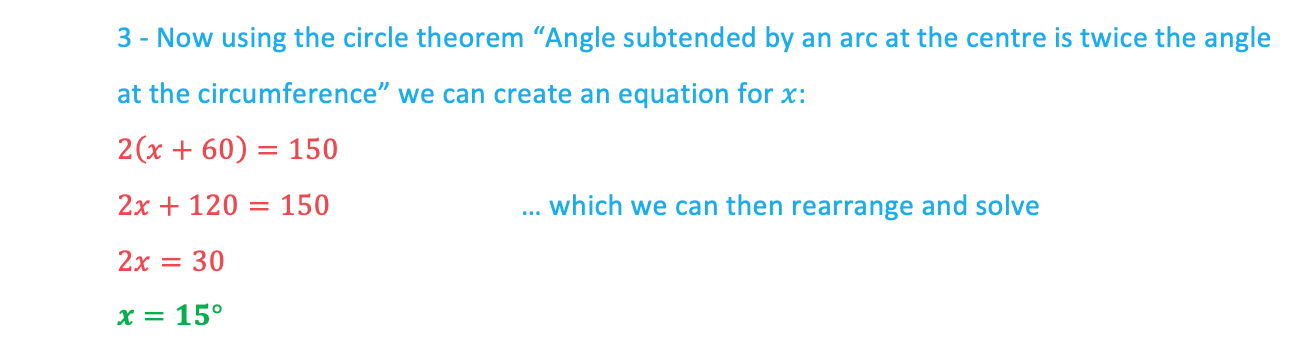#### Worked Example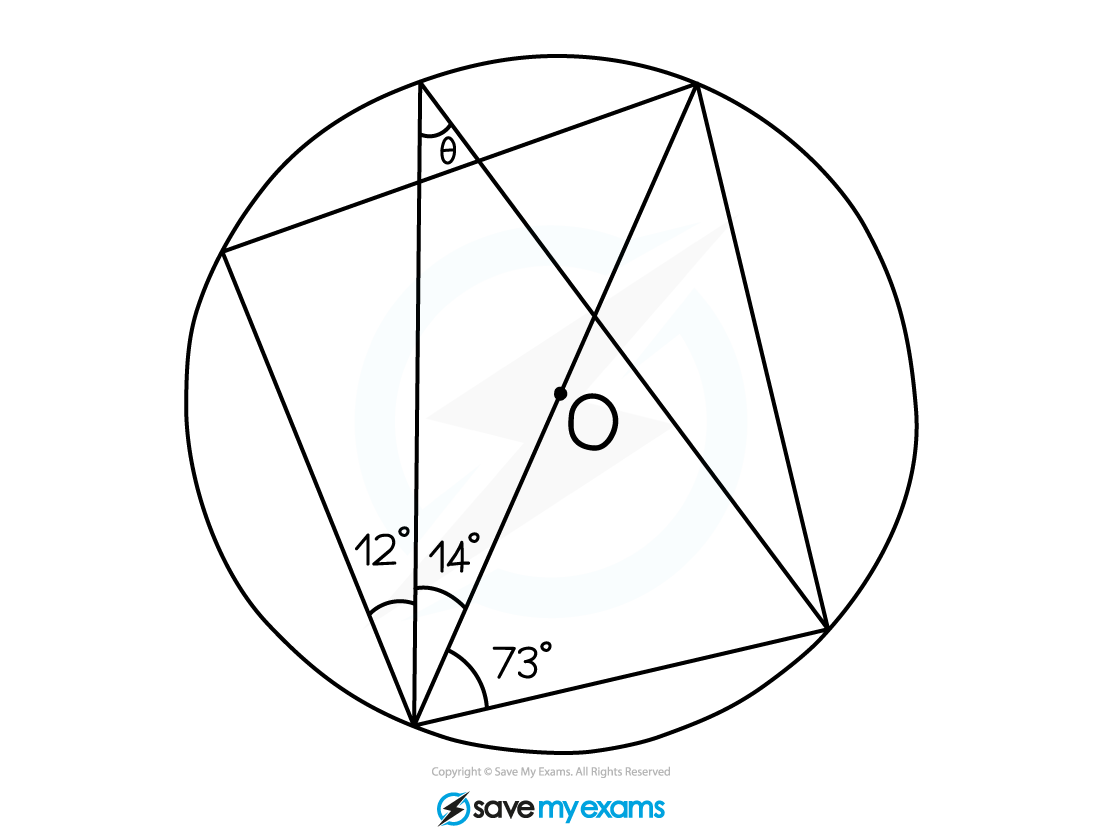#### Worked Example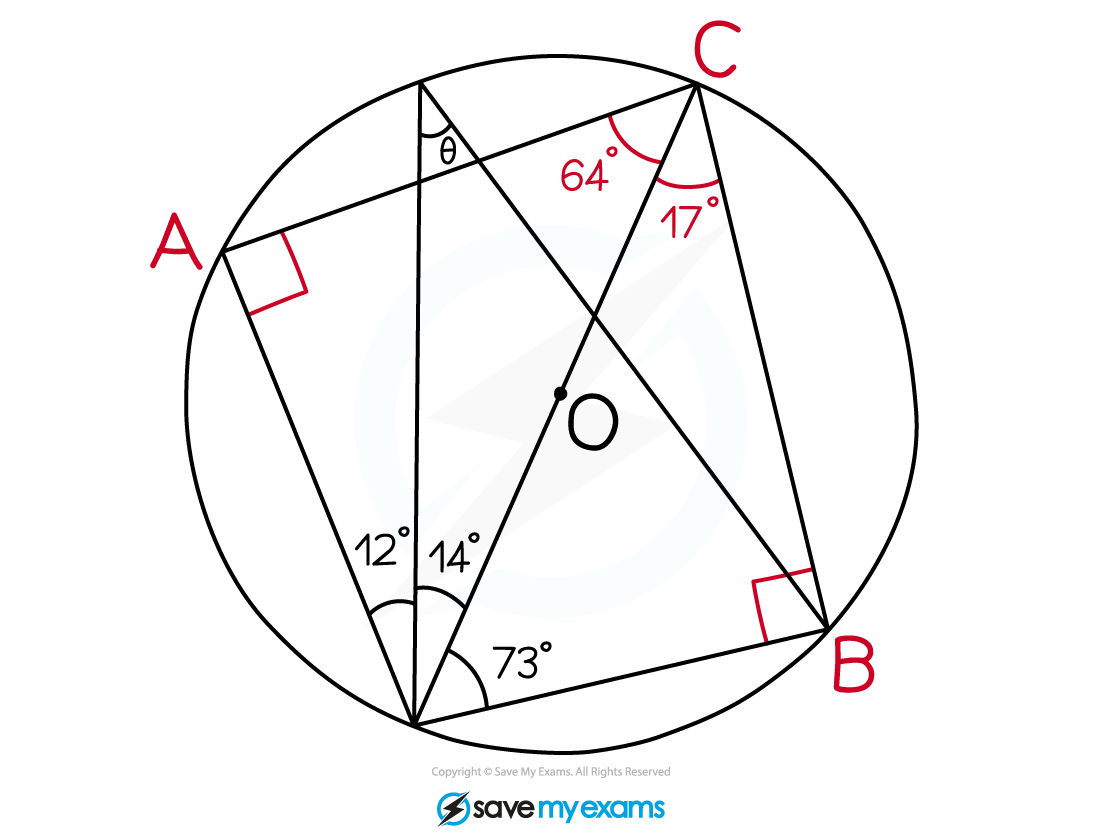#### Worked Example